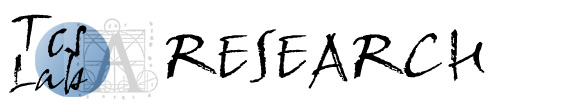### Area I. Constraint satisfaction problems

CSPs are problems defined as a set of variables subject to constraints or restrictions, and the goal is to find an assignment that simultaneously satisfy all constraints. There is a vast number of important problems from many different application areas that can be modelled as CSPs. Prominent examples can be found in circuit design, diagnosis of technical systems, and computer vision.

• Infinite-domain CSPs/Temporal and spatial reasoning
• Nonmonotonic logics
• Counting problems
• Structurally restricted problems

### Area II. Combinatorial optimisation

A branch of optimization where the set of feasible solutions is discrete or can be reduced to a discrete one, and the goal is to find the best possible solution according to some criteria. Well-known examples are the travelling salesman problem, aircrew rostering, and communication network optimisation.

• Generalisations of integer programming
• Submodular optimisation
• Approximation theory

### Area III. Superpolynomial algorithms

Complexity theory teaches us that there are problems that are unlikely to have polynomial-time algorithms. This is no reason for giving up since there is plenty of room for improvements; the difference between an algorithm running in O(22^n) time and an algorithm running in O(1.001^n) time is huge.

• Algorithms for NP- and #P-hard problems
• Methods for analysing algorithms
• Automated construction of algorithms

### Area IV. Automated planning and scheduling

A subarea of computer science and artificial intelligence that concerns the creation of strategies or action sequences for obtaining certain goals, typically for execution by entities such as autonomous robots or unmanned vehicles. Examples of automatic planners include SPSS and Spike for the Hubble space telescope.

• Efficient planning via structural restrictions
• Reactive planning
• Parameterised planning algorithms

### Area V. Combinatorics

A branch of discrete mathematics concerning the study of finite or countable structures. It is related to many other areas of mathematics such as algebra, probability theory, and geometry, as well as to many applied subjects within computer science. For instance, combinatorics is an important ingredient in coding theory, data compression, and cryptography.

• Skolem-like sequences
• Graph theory
• Applications of algebraic geometry

Page responsible: Ulf Nilsson
Last updated: 2012-05-07